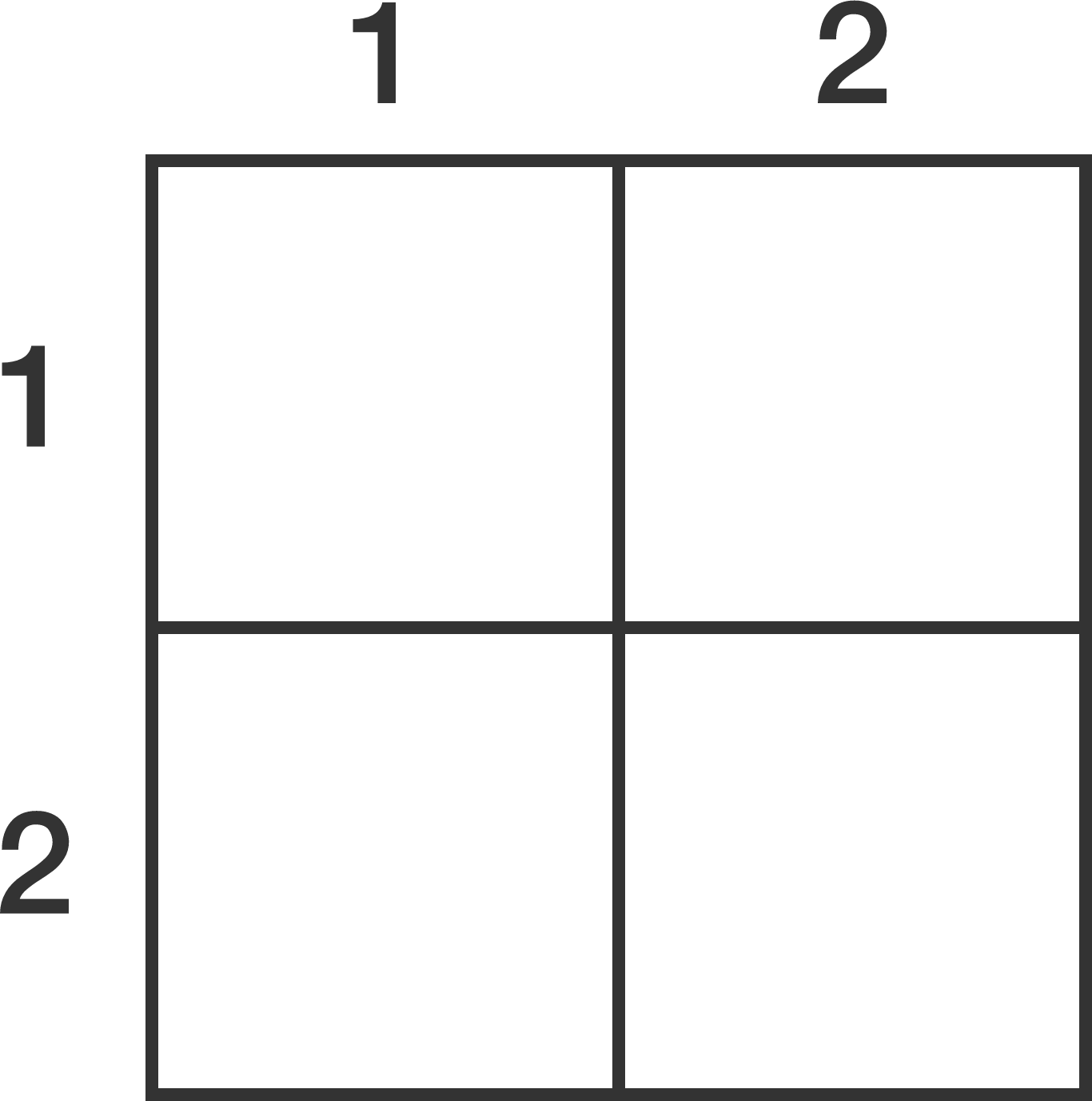# Number Grid PuzzleSolve this mathematics cross number grid puzzle by placing non-zero digits in each cell.

Across
1. Prime
2. Perfect Square

Down
1. Perfect Square
2. Two times 1 Across

What is the sum of all 4 digits?

×